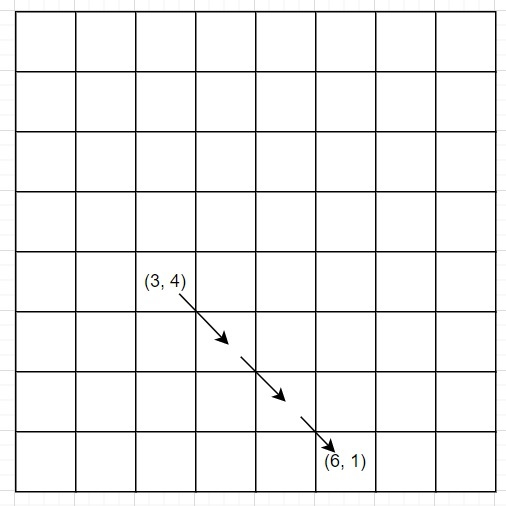# C++ code to count steps to reach final position by robot

Suppose we have two coordinates (x1, y1) and (x2, y2). A robot is at the point (x1, y1) and wants to go to the point (x2, y2). In a single step, the robot can move towards one cell to its 8 adjacent coordinates. We have to find minimal number of steps needed to reach the final position.

So, if the input is like x1 = 3; y1 = 4; x2 = 6; y2 = 1;, then the output will be 3, because## Steps

To solve this, we will follow these steps −

return maximum of |x2 - x1| and |y2 - y1|

## Example

Let us see the following implementation to get better understanding −

#include <bits/stdc++.h>
using namespace std;
int solve(int x1, int y1, int x2, int y2){
return max(abs(x2 - x1), abs(y2 - y1));
}
int main(){
int x1 = 3;
int y1 = 4;
int x2 = 6;
int y2 = 1;
cout << solve(x1, y1, x2, y2) << endl;
}

## Input

3, 4, 6, 1

## Output

3